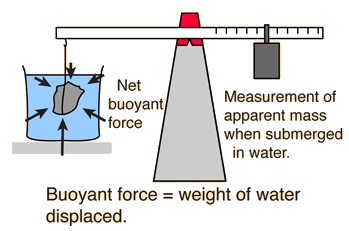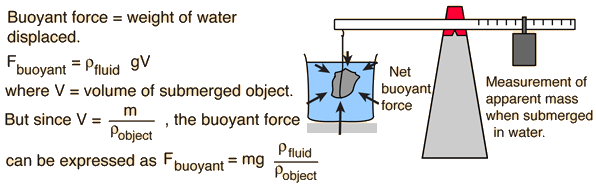# Buoyant ForceSome of the useful applications of buoyancy and Archimedes' principle are to the experimental determination of density.
 Determination of density by water immersion. Determination of density by immersion in a general liquid. Determination of liquid density by immersion of a standard object.
Index

Buoyancy concepts

 HyperPhysics***** Mechanics ***** Fluids R Nave
Go Back

# Buoyant Force, General FluidIf an object is massed in air and found to have mass m = grams

and is then submerged in a fluid and found to have apparent mass m' = grams

then it has displaced m - m' = grams of the fluid. If the density of the fluid is gram/cm3, this implies
Volume of object = V = cm3.
 The density of the object is then ρ = m/V = gm/cm3.

This calculation assumes that the density of the liquid is known, and allows you to change mass, apparent mass, volume or density to explore variations. If you wish to determine the liquid density, then go to fluid density determination. Use of a liquid other than water is sometimes useful, but the most common experimental procedure is immersion in water for density determination, as in the classic Archimedes story.

Note that in the experimental arrangement, the container of fluid in which the object is submerged is supported on a platform so that the balance scale does not include the measurement of the mass of the water and its container.
Index

Buoyancy concepts

 HyperPhysics***** Mechanics ***** Fluids R Nave
Go Back